# Math Fraction Games

Do you want to play fun and interactive math fraction games? The list of games on this webpage is designed to reinforce basic skills, while making learning a breeze.

The math games on this page are suitable for elementary and middle school students.Factors Millionaire Game

Before adding and subtracting fractions, students must understand basic facts about factors and divisibility. This fun game can be used to reinforce these important math concepts.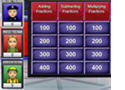Factors and Multiples - Jeopardy Game

In this interactive jeopardy game, students will practice finding the greatest common factor (GCF) and the least common multiple (LCM) of two or more numbers.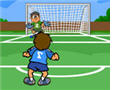Soccer Math Game - Simplifying Fractions

In this fun soccer game, students will practice reducing fractions to the simplest form.Baseball Math Game - Simplifying Fractions

This is a fun baseball math game about game about simplifying fractions. Students will have to first hit a homerun to be able to see and answer a math problem.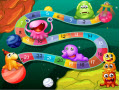Adding and Subtracting Fractions Board Game

Have fun adding and subtracting fractions by playing this interactive board game against another player, or in two teams. This game is an excellent review activity that promotes student engagement.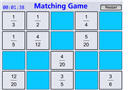Equivalent Fractions Game

In this concentration game, students click on two cards to match equivalent fractions. If there is a match, the problems remain on the page; if not, the cards are turned over.Football Math Game - Adding Fractions

In this game, students will practice adding and subtracting fractions with common and different denominators.Changing Fractions and Decimals to Percents

Young students can use this fun millionaire game to practice changing decimals and fractions to percents.Fractions, Decimals, Percents

In this jeopardy game, students will convert fractions to decimals and percents and vice-versa.Multiplying Fractions Game

In this fun and interactive soccer game, students will test their ability to multiply different fractions.Multiplying Fractions Millionaire Game

How many points can you earn? Practice multiplying fractions by playing this fun game alone, with a partner, or in two teams.Soccer Math - Dividing Fractions Game

Do you know how to divide fractions? Show your friends how many points you can score when playing this fun game.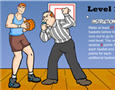Math Basketball - Dividing Fractions Game

Dividing fractions does not have to be boring anymore. Play this fun and interactive game and score as many points as possible.Fractions Jeopardy Game

This online jeopardy game is is a fun way to review the four operations with fractions: addition, subtraction, multiplication, and division.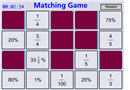Changing Fractions to Percents

In this concentration game, students will match different fractions with the equivalent percents.This Fraction Jeopardy Game has two rounds and three categories: fractions, decimals, and percents. The points are doubled in round two. Have fun earning points!

Return from Math Fraction Games to Math Play homepage.